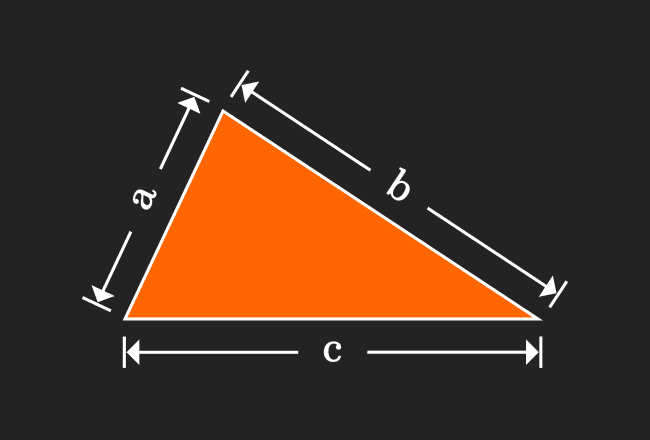# Heron’s formula

## Formula

$A = \sqrt{s(s-a)(s-b)(s-c)}$

### Introduction

A mathematician Heron (or Hero) of Alexandria derived a geometrical proof to express area of a triangle in algebraic form in terms of lengths of three sides and half-perimeter of the triangle. Hence, this formula is called as Heron’s formula or Hero’s formula.$a$, $b$ and $c$ are lengths of three sides of a triangle and its perimeter is denoted by $2s$.

$2s \,=\, a+b+c$

$\implies$ $s \,=\, \dfrac{a+b+c}{2}$

The area of the triangle is denoted by either $A$ or $\Delta$.

$\Delta = \sqrt{s(s-a)(s-b)(s-c)}$

This formula in algebraic form is called as Hero’s (or Heron’s) formula in geometry.

#### Example

Find area of a triangle, if $a = 5cm$, $b = 6cm$ and $c = 7cm$.

Firstly, find the semi perimeter of the triangle.

$s = \dfrac{5+6+7}{2}$

$\implies$ $s = \dfrac{18}{2}$

$\implies$ $\require{cancel} s = \dfrac{\cancel{18}}{{2}}$

$\implies$ $s = 9cm$

Now, find the area of the triangle.

$A = \sqrt{9(9-5)(9-6)(9-7)}$

$\implies$ $A = \sqrt{9(4)(3)(2)}$

$\implies$ $A = \sqrt{9 \times 4 \times 3 \times 2}$

$\implies$ $A = \sqrt{36 \times 6}$

$\,\,\, \therefore \,\,\,\,\,\,$ $A = 6\sqrt{6} \, cm^2$

### Proof

Learn how to derive the hero’s formula in geometrical approach to find the area of a triangle.

Latest Math Topics
Jun 26, 2023
Jun 23, 2023

Latest Math Problems
Jul 01, 2023
Jun 25, 2023
###### Math Questions

The math problems with solutions to learn how to solve a problem.

Learn solutions

Practice now

###### Math Videos

The math videos tutorials with visual graphics to learn every concept.

Watch now

###### Subscribe us

Get the latest math updates from the Math Doubts by subscribing us.